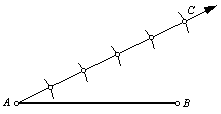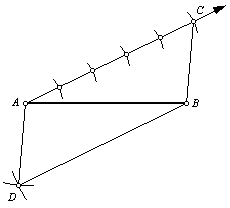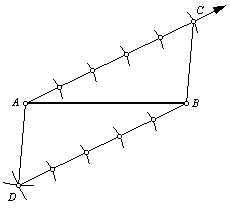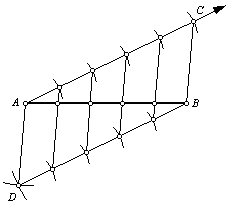Divide a line segment into n congruent line segments.

In this example, n = 5.

 1. Begin with line segment AB. It will be divided into five congruent line segments.2. Draw a ray from point A. Use the compass to step off five uniformly spaced points along the ray. Label the last point C.3. Draw an arc with the compass centered at point A, with radius BC. Draw a second arc with the compass centered at point B, with radius AC. Label the intersection point D. Note that ACBD is a parallelogram.4. Use the compass to step off points along line segment DB, using the same radius that was used for the points along line segment AC.5. Use the straightedge to connect the corresponding points. These line segments will be parallel. They cut line segments AC and DB into congruent segments. Therefore, they must also cut line segment AB into congruent segments.Back to Geometry Construction Reference